﻿ 基于双目立体视觉和形变模型的人脸三维重建研究 Research on 3D Reconstruction of Face Based on Binocular Stereo Vision and Deformation Model

Journal of Image and Signal Processing
Vol.07 No.03(2018), Article ID:26014,9 pages
10.12677/JISP.2018.73019

Research on 3D Reconstruction of Face Based on Binocular Stereo Vision and Deformation Model

Xuhao Xiao1, Junjie Li1, Haihui Wang1,2*, Qing Hou1, Zhi Ruan1

1School of Computer Science and Engineering, Wuhan Institute of Technology, Wuhan Hubei

2Hubei Provincial Key Laboratory of Intelligent Robot, Wuhan Institute of Technology, Wuhan Hubei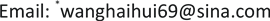Received: Jun. 28th, 2018; accepted: Jul. 12th, 2018; published: Jul. 20th, 2018ABSTRACT

People usually perceive things from a three-dimensional perspective, requiring the computer to automatically reconstruct the corresponding three-dimensional shape model using two-dimensional human face images. The three-dimensional face reconstruction based on 3DMM under binocular vision is to use SIFT algorithm for feature point matching and disparity calculation to obtain three-dimensional coordinates. The three-dimensional coordinates are applied directly to the face deformation model, which overcomes the traditional method of finding two-dimensional and three-dimensional coordinates, convert the relationship, the disadvantage of low precision, thus reconstruct a three-dimensional face model with a strong sense of reality.

Keywords:Face Detection, Three-Dimensional Reconstruction, SIFT Algorithm, Deformation Model

1武汉工程大学计算机科学与工程学院，湖北 武汉

2武汉工程大学智能机器人湖北省重点实验室，湖北 武汉Copyright © 2018 by authors and Hans Publishers Inc.1. 引言

2. 系统组成

3. 识别算法与重建

3.1. 匹配算法

SIFT算法是David Lowe于1999年提出的基于局部区域的特征描述子，能同时解决两幅图像之间发生的各种任意的旋转和平移，以及不同角度的仿射变换等情况下的匹配问题；另外SIFT扩展性强，可以很方便的与其他形式的特征向量进行联合  。

SIFT特征匹配分为三个工序：提取关键点，生成特征描述器，特征点匹配。SIFT算法先求解图像每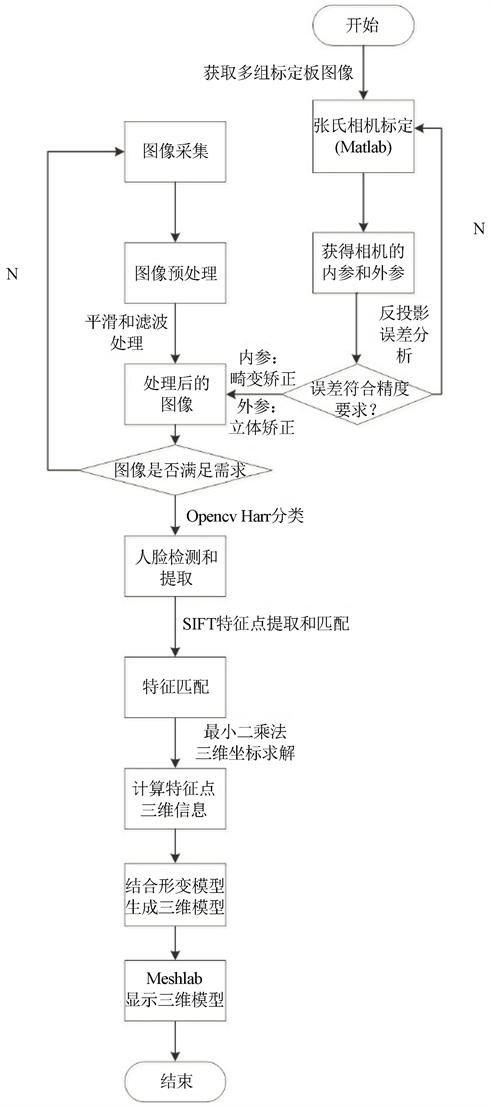Figure 1. System composition

$g=\sqrt{{g}_{x}^{2}+{g}_{y}^{2}}$ (1)

$\theta =\mathrm{arctan}\frac{{g}_{y}}{{g}_{x}}$ (2)

${R}_{i}=\left({r}_{i1},{r}_{i2},\cdots ,{r}_{i128}\right)$ 和实时图的关键点描述子 ${S}_{i}=\left({s}_{i1},{s}_{i2},\cdots ,{s}_{i128}\right)$  ，然后计算任意两描述子相识性度量

$d\left({R}_{i},{S}_{i}\right)=\sqrt{{\sum }_{j=1}^{128}{\left({r}_{ij}-{s}_{ij}\right)}^{2}}$ (3)

SURF构造的金字塔图像与SIFT有非常大的不同点。SIFT使用的是DOG特征，而SURF  使用的是Hessian矩阵行列式中的近似值图像，使得检测速度变快。图像中某个像素点的Hessian矩阵如下：

$H\left(f\left(x,y\right)\right)=\left[\begin{array}{cc}\frac{{\partial }^{2}f}{\partial {x}^{2}}& \frac{{\partial }^{2}f}{\partial x\partial y}\\ \frac{{\partial }^{2}f}{\partial x\partial y}& \frac{{\partial }^{2}f}{\partial {y}^{2}}\end{array}\right]$ (4)

$H\left(x,\sigma \right)=\left[\begin{array}{cc}{L}_{xx}\left(x,\sigma \right)& {L}_{xy}\left(x,\sigma \right)\\ {L}_{xy}\left(x,\sigma \right)& {L}_{yy}\left(x,\sigma \right)\end{array}\right]$ (5)

${S}_{x}=S\left({X}_{1},{Y}_{1}\right)+S\left({X}_{4},{Y}_{4}\right)-S\left({X}_{2},{Y}_{2}\right)-S\left({X}_{3},{Y}_{3}\right)$ (6)

$\mathrm{det}\left({H}_{approx}\right)={D}_{xx}{D}_{yy}-{\left(0.9{D}_{xy}\right)}^{2}$ (7)

3.2. 三维坐标求解算法

$\begin{array}{l}{X}_{w}=\frac{{Z}_{w}}{f}{v}_{1}=\frac{{u}_{1}B}{d}\\ {Y}_{w}=\frac{{Z}_{w}}{f}{v}_{1}=\frac{{v}_{1}B}{d}\\ {Z}_{w}=\frac{fB}{d}\end{array}$ (8)

3.3. 人脸三维重建算法

Opencv的人脸检测主要通过Haar分类器实现，预先训练好物体的检测器(xml格式)，包括正脸、侧脸、眼睛等特征。大概思路是首先将图像均衡归一化到同样大小，利用多级高检测率低拒绝率的多层树分类器逐步拒绝所有的非人脸部分，最后剩下的就是人脸区域。将人其标记截取出来。

${S}_{new}={\sum }_{i=1}^{m}{a}_{i}{S}_{i}={a}_{1}{S}_{1}+{a}_{2}{S}_{2}+\cdots +{a}_{m}{S}_{m}$ (9)

${a}_{i}$ 为组合因子，满足 ${\sum }_{i=1}^{m}{a}_{i}=1$

${S}_{i}={\left({x}_{1},{y}_{1},{z}_{1},{x}_{2},{y}_{2},{z}_{2},\cdots ,{x}_{k},{y}_{k},{z}_{k}\right)}^{\text{T}}$ (10)

${S}_{new}=\overline{S}+P\beta$ (11)

$\text{E}\left(\beta \right)={‖{P}^{f}\beta -\Delta d‖}^{2}+\eta {‖\beta ‖}^{2}$ (12)

$\text{argmin}‖\text{E}\left(\beta \right)‖=V\left(\frac{{\lambda }_{i}}{{\lambda }_{i}^{2}+\eta }\right){U}^{\text{T}}\text{Δ}d$ (13)

${S}_{new}=\overline{S}+PV\left(\frac{{\lambda }_{i}}{{\lambda }_{i}^{2}+\eta }\right){U}^{\text{T}}\Delta d$ (14)

4. 实验结果与分析

4.1. 特征匹配Table 1. Three-dimensional information of five sets of feature points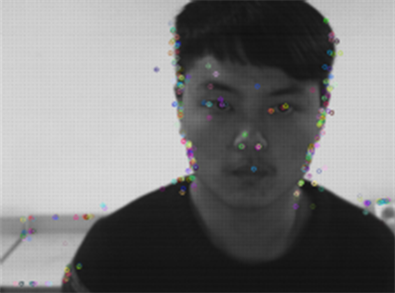Figure 2. Results of feature point extraction by SIFT

4.2. 三维坐标求解

4.3. 人脸三维重建

5. 结语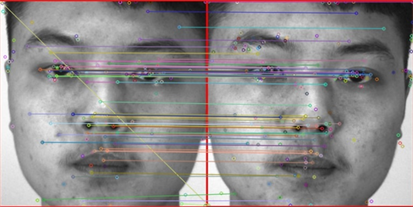Figure 3. Results of feature matching by SIFT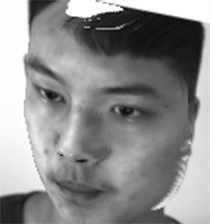Figure 4. Results of 3D reconstruction of the faceTable 2. Contrast results of 3D reconstruction of face and time of use

Research on 3D Reconstruction of Face Based on Binocular Stereo Vision and Deformation Model[J]. 图像与信号处理, 2018, 07(03): 161-169. https://doi.org/10.12677/JISP.2018.73019

1. 1. Zhang, Q. and Pless, R. (2014) Extrinsic Calibration of a Camera and Laser Range Finder (Improves Camera Ealibration). Proceedings of 2014 IEEE/RSJ International Conference on Intelligent Robots and Systems (IROS 2014), Sendai, 28 September-2 October 2004.

2. 2. Liao, H.B., Chen, Q.H., Zhou, Q.J., et al. (2012) Nonuniform Resampling Based on Method for Pixel-Wise Correspond-ence between 3D Faces. Journal of Visual Communication and Image Representation, 23, 924-931.
https://doi.org/10.1016/j.jvcir.2012.06.005

3. 3. Patel, A. and Smith, W.A.P. (2012) Driving 3D Morphable Models Using Shading Cues. Pattern Recognition, 45, 1993-2004.
https://doi.org/10.1016/j.patcog.2011.11.013

4. 4. Kemelmacher-Shlizerman, I. and Basri, R. (2011) 3D Face Reconstruction from a Single Image. Using a Single Reference Face Shape. IEEE Transactions on Pattern Analysis and Machine Intelligence, 3.

5. 5. Patel, A. and Smith, W.A.P. (2012) Driving 3D Morphable Models Using Shading Cues. Pattern Recognition, 45, 1993-2004.

6. 6. 谷天, 陈江龙, 李科, 等. 一种用于三维人脸重建的快速稠密视差图生成方法[J]. 西华大学学报(自然科学版), 2016, 35(1): 77-79.

7. 7. 隋巧燕, 董洪伟, 刘蕾. 双目下点云的三维人脸重建[J]. 现代电子技术, 2015(4): 102-105.

8. 8. 鹿乐, 鞠园, 蔡亮, 等. 基于特征分块的快速三维人脸重建[J]. 火力与指挥控制, 2014(z1): 152-155.

9. 9. 孙曦. 基于双目立体视觉的人脸三维重建与识别[D]: [博士学位论文]. 北京: 中国科学技术大学, 2011.

10. 10. 王小文. 基于形变模型的多摄像机人脸三维重建[D]: [硕士学位论文]. 北京: 北京理工大学, 2010.

11. 11. Xu, G. and Zhang, Z. (2013) Epipolaf Geometry in Stereo, Motion and Object Recognition: Aunified Approach. Springer Science & Business Media, Berlin.

12. 12. 吴子扬, 周大可, 杨欣, 等. 由粗到精的三维人脸稀疏重建方法[J]. 吉林大学学报(信息科学版), 2013, 31(6): 582-588.
https://doi.org/10.3969/j.issn.1671-5896.2013.06.005

NOTES

*通讯作者。